# Research on the Influence of Solid Volume Fractions on Turbine Performance

Research on the Influence of Solid Volume Fractions on Turbine Performance

Huiyan Wang

Key Laboratory of Fluid and Power Machinery (Xihua University), Ministry of Education, Chengdu 610039, China

Corresponding Author Email:
why@mail.xhu.edu.cn
Page:
630-636
|
DOI:
https://doi.org/10.18280/ijht.340412
|
Accepted:
|
Published:
31 December 2016
| Citation

OPEN ACCESS

Abstract:

The existence of sediment in water causes excessive abrasion of turbine blades and affects the performance of the turbine. It is important to predict the influence of solid volume fractions on turbine performance. The solid-liquid two-phase turbulent flow in a Francis turbine was numerically simulated by establishing a mathematical model for the flow passage of the turbine on the basis of the time-averaged basic equations and κ-ε equations. The turbulent flow in this turbine was calculated on the design point in clear water, as well as in sandy water with the average solid volume fractions of 0.5 % and 5 % at the inlet of the spiral case. The pressure distributions and sand concentrations on the leading side and suction side of the runner blades, as well as the velocities, turbulent kinetic energies and their dissipation rates on the horizontal section of runner were compared under these three conditions to illustrate the influences of the solid volume fractions on the turbine performance. The results show that with increasing solid volume fraction, the pressure difference on the leading side and suction side of the blade increased; the sand erosion on the leading side was much worse than that on the suction side, especially at the outlet area near the runner band; the velocity changes of sand particles and water were small at the outlet area, and the velocity of sand particles became smaller than that of water; the turbulent kinetic energy and its dissipation rate were slightly affected by the solid volume fractions. All of these factors lead to serious cavitation on the suction side and sand erosion on the leading side. The joint effect of cavitation and sand erosion will be aggravated with increasing solid volume fractions which will further reduce the performance of the turbine.

Keywords:

Francis turbine, Pressure distribution, Solid volume fraction, Turbulent flow, Velocity distribution.

1. Introduction

In recent years, the solid volume fraction of most rivers has become higher and higher due to the deterioration of the ecological environment. Hence, it has been necessary to use water resources with large solid volume fractions to generate power. The presence of sand particles in water often causes excessive abrasion of turbine components exposed to the flow . In particular, the flow components of Francis turbines are the most vulnerable to abrasive damage . The abrasion of these components may result in considerable reduction of the performance of the entire power plant . It can also lead to a reduced service life which in turn reduces the time between overhauls. The higher frequency of overhaul also decreases the total energy production. Most of the engineering designs are based on the design theory in clear water, and then corrected to the sandy water conditions. However, the performance of the turbine cannot be guaranteed in water with large solid volume fractions. A prototype experiment can solve this problem but with a great cost. Therefore, the numerical simulation  of solid-liquid two-phase flow in Francis turbines has become an important basis for the study of sand abrasion of the turbine [5, 6]. This method has been proved reliable and accurate . Due to the complicated flow state in a Francis turbine, especially the multi-phase turbulent flow, it is necessary to carry out in-depth study of the flow in Francis turbines to find out what is going on inside the turbine, and to predict the performance of the turbine running on rivers with large solid volume fractions. Presently, the CFD method has been used to study the three-dimensional turbulent flow field in hydraulic machinery, and scholars [8, 9] have used this method to calculate the solid phase flow in hydraulic machinery. Takagi et al.  reported on the hydraulic performance tests on a Francis turbine model with sediment laden flow, conducted in Japan, and showed that the turbine’s best efficiency decreased in direct proportion to the increase in solids concentration. Keck et al.  presented a study on the utilization of the CFD method to predict the erosion pattern in a hydraulic turbine and compared it with field measurements of the erosion. P. J. Dunstan  studied the enhancement of cavitation by silt erosion. Schilling R.  simulated the two-phase flow in centrifugal pump impellers. S. Chitrakar  studied the simultaneous effects of secondary flow and sediment erosion in Francis turbines. Eltvik M. et al.  investigated the relationships between sediment erosion and the operating conditions of the turbine, and found that the erosion process was strongly dependent on the operating conditions of the turbines. In this work, a mathematical model was established for the entire flow passage of a Francis turbine, and then the flow conditions in the turbine runner were simulated to understand the influences of the solid volume fraction on turbine performance.

2. Mathematical Model

The following assumptions have been made in this study:

1. The solid phase consists of sand particles spherical in shape and uniform in size.
2. The liquid phase (water) is incompressible. The solid phase (sand) is continuous. The physical properties of each phase are constants.
3. Neither the suspended matter nor the liquid has any phase changes.
4. Interactions between particles, as well as between particles and the wall are neglected.

The usual approach for describing turbulence transport in the flow is to separate each of the instantaneous variables into a mean part and a fluctuating part. Thus, the time-averaged continuity equations and momentum equations for solid-liquid two-phase flows can be written as :

Time-averaged liquid phase continuity equation:

$\frac{\partial \varphi_{f}}{\partial t}+\frac{\partial}{\partial x_{i}}\left(\varphi_{f} V_{f i}-\frac{v_{t}}{\sigma_{\varphi f}} \frac{\partial \varphi_{f}}{\partial x_{i}}\right)=0$(1)

Time-averaged solid phase continuity equation:

$\frac{\partial \varphi_{s}}{\partial t}+\frac{\partial}{\partial x_{i}}\left(\varphi_{s} V_{s i}-\frac{v_{t}}{\sigma_{\varphi s}} \frac{\partial \varphi_{s}}{\partial x_{i}}\right)=0$(2)

Time-averaged liquid phase momentum equation:

$\frac{\partial }{\partial t}\left( {{\varphi }_{f}}{{V}_{fi}} \right)+\frac{\partial }{\partial {{x}_{j}}}({{\varphi }_{f}}{{V}_{fi}}{{V}_{fj}})$$=\frac{\partial }{\partial {{x}_{j}}}\left[ \frac{{{\nu }_{t}}}{{{\sigma }_{\varphi f}}}\left( {{V}_{fi}}\frac{\partial {{\varphi }_{f}}}{\partial {{x}_{j}}}+{{V}_{fj}}\frac{\partial {{\varphi }_{f}}}{\partial {{x}_{i}}} \right)-{{\varphi }_{f}}\overline{{{\upsilon }_{fi}}{{\upsilon }_{fj}}} \right]$$-\frac{1}{{{\rho }_{f}}}\left( {{\varphi }_{f}}\frac{\partial p}{\partial {{x}_{i}}}+\overline{{{\phi }_{f}}\frac{\partial p'}{\partial {{x}_{i}}}} \right)+{{\nu }_{f}}\frac{\partial }{\partial {{x}_{j}}}\left[ {{\varphi }_{f}}\left( \frac{\partial {{V}_{fi}}}{\partial {{x}_{j}}}+\frac{\partial {{V}_{fj}}}{\partial {{x}_{i}}} \right) \right]$$-\frac{B}{{{\rho }_{f}}}\left[ {{\varphi }_{f}}{{\varphi }_{s}}\left( {{V}_{fi}}-{{V}_{si}} \right)+\left( {{\varphi }_{s}}-{{\varphi }_{f}} \right)\left( \frac{{{\nu }_{t}}}{{{\sigma }_{\varphi s}}}-\frac{{{\nu }_{t}}}{{{\sigma }_{\varphi f}}} \right)\frac{\partial {{\varphi }_{f}}}{\partial {{x}_{i}}} \right]+{{\varphi }_{f}}{{g}_{i}} (3) Time-averaged solid phase momentum equation: \frac{\partial}{\partial t}\left(\varphi_{s} V_{s i}\right)+\frac{\partial}{\partial x_{j}}\left(\varphi_{s} V_{s i} V_{s j}\right)$$=\frac{\partial}{\partial x_{j}}\left[\frac{v_{t}}{\sigma_{\varphi s}}\left(V_{s i} \frac{\partial \varphi_{s}}{\partial x_{j}}+V_{s j} \frac{\partial \varphi_{s}}{\partial x_{i}}\right)-\varphi_{s} \overline{v_{s i} v_{s j}}\right]$$-\frac{1}{\rho_{s}}\left(\varphi_{s} \frac{\partial p}{\partial x_{i}}-\overline{\varphi_{f}} \frac{\partial p^{\prime}}{\partial x_{i}}\right)+v_{s} \frac{\partial}{\partial x_{j}}\left[\varphi_{s}\left(\frac{\partial V_{s i}}{\partial x_{j}}+\frac{\partial V_{s j}}{\partial x_{i}}\right)\right]$$-\frac{B}{\rho_{s}}\left[\phi_{f} \phi_{s}\left(V_{s i}-V_{f i}\right)+\left(\phi_{f}-\phi_{s}\right)\left(\frac{v_{t}}{\sigma_{\phi s}}-\frac{v_{t}}{\sigma_{\phi f}}\right) \frac{\partial \phi_{s}}{\partial x_{i}}\right]+\phi_{s} g_{i}$(4)

The turbulent kinetic energy equation (k equation) for solid-liquid two-phase flow is:

$\frac{\partial }{\partial t}\left( {{\varphi }_{f}}k \right)+\frac{\partial }{\partial {{x}_{j}}}({{\varphi }_{f}}{{V}_{fj}}k)=\frac{\partial }{\partial {{x}_{j}}}\left( \frac{{{\nu }_{t}}}{{{\sigma }_{\varphi f}}}{{\varphi }_{f}}\frac{\partial k}{\partial {{x}_{j}}} \right)-{{\varphi }_{f}}\varepsilon +{{P}_{r}}+{{V}_{Df}}+{{A}_{Ds}}$ (5)

The turbulent energy dissipation rate equation (ε equation) for solid-liquid two-phase flow is:

$\frac{\partial}{\partial t}\left(\varphi_{f} \varepsilon\right)+\frac{\partial}{\partial x_{j}}\left(\varphi_{f} V_{f j} \varepsilon\right)$$=\frac{\partial}{\partial x_{j}}\left[\left(v_{f}+\frac{v_{t}}{\sigma_{\varepsilon}}\right) \phi_{f} \frac{\partial \varepsilon}{\partial x_{j}}\right]+\left[C_{\varepsilon 1} P_{r}-\phi_{f}\left(C_{\varepsilon 2} \varepsilon+C_{\varepsilon 3} A_{D s}\right)\right] \frac{\varepsilon}{k}(6) where, \overline{\phi_{f}} \frac{\partial p^{\prime}}{\partial x_{i}}=-\rho_{f} \frac{\partial}{\partial x_{i}}\left[\left(C_{1} k^{1 / 2}+C_{2} \frac{k^{3 / 2}}{\varepsilon} \frac{\partial V_{f i}}{\partial x_{k}}\right) \frac{\nu_{t}}{\sigma_{\phi f}} \frac{\partial \phi_{f}}{\partial x_{k}}\right]$$+\rho_{f} \frac{\varepsilon}{k}\left[C_{3} \overline{\phi_{f} v_{f i}}+C_{4}\left(\frac{1}{k} \overline{v_{f h} v_{j k}}+\frac{2}{3} \delta_{i k} \frac{v_{t}}{\sigma_{\varphi f}} \frac{\partial \varphi_{f}}{\partial x_{k}}\right)\right]$$-\rho_{f}\left(C_{5} \frac{\partial V_{f i}}{\partial x_{k}}+C_{6} \frac{\partial V_{f k}}{\partial x_{i}}\right) \frac{v_{t}}{\sigma_{\varphi f}} \frac{\partial \varphi_{f}}{\partial x_{i}} p_{r}=\frac{\partial}{\partial x_{j}}\left(\frac{v_{t}}{\sigma_{\phi f}} V_{f i} V_{f j} \frac{\partial \phi_{f}}{\partial x_{i}}-V_{f i} \overline{\varphi_{f} v_{f i} v_{f j}}-V_{f j} \overline{\varphi_{f} v_{f i}^{2}}\right)$$-\frac{\partial}{\partial x_{j}}\left(\phi_{f} V_{f i}\right) \overline{v_{f i} v_{f j}}+\frac{1}{\rho_{f}} \frac{v_{t}}{\sigma_{\phi f}} \frac{\partial \phi_{f}}{\partial x_{j}} \frac{\partial p}{\partial x_{j}}$$-C_{k} \frac{v_{t}}{\sigma_{\varphi f}} \frac{k}{\varepsilon}\left(V_{f i} \frac{\partial \varphi_{f}}{\partial x_{j}}+V_{f j} \frac{\partial \varphi_{f}}{\partial x_{i}}\right) V_{D f}=v_{f}\left\{\phi_{f} \frac{\partial^{2} k}{\partial x_{j}^{2}}+\frac{\partial \phi_{f}}{\partial x_{j}}\left(\frac{\partial k}{\partial x_{j}}+\frac{\partial \overline{v_{f i} U_{f j}}}{\partial x_{i}}+v_{t} \frac{\partial^{2} V_{f i}}{\partial x_{j}^{2}}\right)\right.$$-\frac{\partial}{\partial x_{j}}\left[\frac{v_{t}}{\sigma_{\varphi f}} \frac{\partial \varphi_{f}}{\partial x_{i}}\left(\frac{\partial V_{f i}}{\partial x_{j}}+\frac{\partial V_{f j}}{\partial x_{i}}\right)\right] \}$

$A_{D S}=-\frac{B}{\rho_{f}}\left[\varphi_{f} \varphi_{s}\left(\overline{v_{f i} v_{f i}}-\overline{v_{f i} v_{s i}}\right)+\frac{v_{t}}{\sigma_{\varphi f}}\left(\varphi_{f}-\varphi_{s}\right)\left(V_{f i}-V_{s i}\right) \frac{\partial \varphi_{f}}{\partial x_{i}}\right]-\frac{v_{t}}{\sigma_{\phi f}} \frac{\partial \phi_{f}}{\partial x_{i}} g_{i}$

$\overline{{{\phi }_{f}}{{\upsilon }_{fi}}{{\upsilon }_{fj}}}={{C}_{k}}\frac{k}{\varepsilon }\left( \overline{{{\upsilon }_{fi}}{{\upsilon }_{fk}}}\frac{\partial }{\partial {{x}_{k}}}\left( \frac{{{\nu }_{t}}}{{{\sigma }_{\varphi f}}}\frac{\partial {{\varphi }_{f}}}{\partial {{x}_{j}}} \right)+\overline{{{\upsilon }_{fj}}{{\upsilon }_{fk}}}\frac{\partial }{\partial {{x}_{k}}}\left( \frac{{{\nu }_{t}}}{{{\sigma }_{\varphi f}}}\frac{\partial {{\varphi }_{f}}}{\partial {{x}_{i}}} \right) \right)$

In the above equations: subscripts f and s represent the liquid and the solid phases, respectively, and i, j, k tensors; t is the time; Vi the time-averaged velocity components; $v_{i}$ the fluctuating velocity components; xi the components of coordinate; P the time-averaged pressure; $p^{\prime}$ the fluctuating pressure; $\phi$  the time-averaged volume fraction (concentration); φ the fluctuating volume fraction; gi the component of gravity acceleration in i direction; ρ the material density; ν the material kinematic viscousity; κ the turbulent kinetic energy of liquid phase where $\kappa=\overline{v_{f i} v_{f i}} / 2$; ε the turbulent energy dissipation rate of liquid phase; B the interphase friction coefficient and B=18(1+B0)ρfνf/d2; and d the particle diameter of solid phase. B0 was introduced in order to consider the effects of the virtual mass force, Saffman force and Magus force etc., except the linear Stokes drag. C1=-1.0, C2=1.0, C3=4.3, C4=-3.2, C5=-0.8, C6=0.2, $\sigma_{\mathcal{E}}$=1.3, $C_{\varepsilon 1}$=1.44, $C_{\varepsilon 2}$=1.92, $C_{\varepsilon 3}$=1.2, and Ck≈0.1.

The mean relation equations for the liquid and solid volume fractions are:

$\varphi_{f}+\varphi_{s}=1, \phi_{f}+\phi_{s}=0$(7)

$\nu_{t}$ is the kinematic eddy viscousity, and $v_{t}=C_{\mu} k^{2} / \varepsilon\left(C_{\mu} \approx 0.09\right)$.

$\mathcal{V}_{t}$ is the turbulent Schmidt number of $\phi$ and $\sigma_{\varphi f} \approx 1 . \sigma_{\varphi s}$ is given by Peskin , and $\sigma_{\varphi_{S}}=\left(1-\frac{T_{L}^{2} \varepsilon}{15 v_{f}} \frac{6 A^{2}}{A+1}\right)^{-1}$.

where, A is the ratio of particle response time $\tau_{p}$ [ρsd2/(18ρfνf)] to the fluid Largangian integral time scale $T_{L}\left(T_{L}=C_{T} k / \varepsilon, \quad C_{T} \approx 0.20-0.41\right)$.

The liquid phase Reynolds stress $\overline{v_{f i} v_{f j}}$ and the solid phase Reynolds stress $\overline{v_{s i} v_{s j}}$ are respectively calculated from:

$\overline{{{\upsilon }_{fi}}{{\upsilon }_{fj}}}=-\frac{{{\nu }_{t}}}{{{\sigma }_{\varphi f}}}\left( \frac{\partial {{V}_{fi}}}{\partial {{x}_{j}}}+\frac{\partial {{V}_{fj}}}{\partial {{x}_{i}}} \right)+\frac{2}{3}{{\delta }_{ij}}\left( k+\frac{{{\nu }_{t}}}{{{\sigma }_{\varphi f}}}\frac{\partial {{V}_{fk}}}{\partial {{x}_{k}}} \right)$

$\overline{{{\upsilon }_{si}}{{\upsilon }_{sj}}}=-\frac{{{\nu }_{t}}}{{{\sigma }_{\varphi s}}}\left( \frac{\partial {{V}_{si}}}{\partial {{x}_{j}}}+\frac{\partial {{V}_{sj}}}{\partial {{x}_{i}}} \right)+\frac{2}{3}{{\delta }_{ij}}\left( {{k}_{s}}+\frac{{{\nu }_{t}}}{{{\sigma }_{\varphi s}}}\frac{\partial {{V}_{sk}}}{\partial {{x}_{k}}} \right)$ (8)

where, $\delta_{i j}$ is Kronecker delta, ks the turbulent kinetic energy of the solid phase, and $k_{s}=\overline{v_{s i} v_{s i}} / 2$. For large flow Reynolds numbers and short particle response time, ks is approximated by k/(A+1). The correlation $\overline{v_{f i} v_{s j}}$is approximated by 2$k /(A+1)$.

This model has been used to simulate the solid-liquid two-phase turbulent flow in the runner of Francis turbines used in Jinping II Hydropower Station on Yalong River in China, and has been proved reliable .

3. Numerical Calculation

3.1 Geometrical model and mesh generation

The turbine simulated was a vertical metal case Francis turbine. The design parameters and flow calculation parameters of this turbine are listed in Table 1.

Table 1. Design parameters of turbine and flow calculation parameters

 Parameters Value Design head, Hp 220 m Design flow, Qd 13.05 m3.s-1 Speed, n 1000 r.min-1 Runner diameter, D1 1080 mm Number of runner blades, Z 19 Number of stay vanes, Z2 9 Number of wicket gates, Z1 16 Height of guide vane, b0 285 mm Diameter of distribution circle, D0 1400 mm Type of guide vane positive curvature guide vane Particle diameter 0.1 mm Sand density 2650 kg.m-3 Water density 1000 kg.m-3

As the geometry of the turbine body is complicated, it was necessary to divide the body into several parts for solid modeling and mesh generation. Firstly, CAD technology and UG NX software were used in this study to establish the digital geometric models of all flow components of the turbine, as shown in Figure 1. Secondly, the ICEM CFD software was used for mesh generation, and the unstructured tetrahedron meshes were used. The function of Mesh Quality in the ICEM CFD software was used to check the quality of generated meshes (the mesh was qualified if the Quality value was more than 0.2), and the function of Smooth Mesh was used to optimize these meshes. Thirdly, the function of Merge in ICEM CFD was used to connect the meshes of all parts, and then the computational meshes of the whole flow passage were obtained, as shown in Figure 2. The total numbers of computational meshes generated for the whole flow passage of the turbine were 1,219,914, 1,917,481 and 2,193,457, and the later two met the independency requirement of less than 5 %. To save the calculation time, the 1,917,481 value was used. This included 479,516 meshes for the turbine case (including a part of the inlet pipe) with a minimum Quality value of 0.363; 323,396 meshes for the stay vane and wicket gate with a minimum Quality value of 0.291; 485,405 meshes for the runner with a minimum quality value of 0.334; and 629,164 meshes for the draft tube with a minimum quality value of 0.311. The Quality values of all meshes in the various components were more than 0.2, so their qualities met the requirements.

## 1.pngFigure 1. Whole flow passage model of the Francis turbine

## 2.png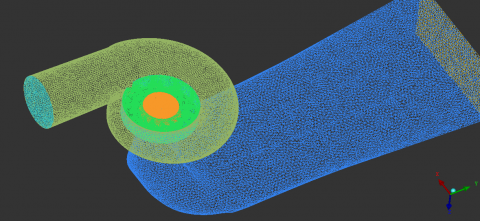Figure 2. Meshes of the whole flow passage

3.2 Numerical method

By comparing Francis turbines with high and medium specific speeds, the parts of the turbine which are severely worn were the outlet tip of the blade near the band and the inner face of the band . So, the velocity distribution and pressure distribution on the runner blades were analyzed in emphasis. This study was based on the time-averaged basic equations and k-ε equations. By using the AEA Technology in CFX software and SIMPLEC algorithm, the turbulent flow in the Francis turbine was calculated on the design point (Qd=13.05 m3/s) in clear water, as well as in sandy water with average solid volume fractions of 0.5 % and 5 % at the inlet of the spiral case with solid-liquid two-phase flow.

3.3 Boundary conditions

The velocity inlet was adopted. It was assumed that the inlet had uniform incoming flow and the inlet velocity was perpendicular to the inlet boundary surface. By using the inlet velocity, we could calculate the values of k and ε. The outlet was provided with the conditions of free development, that is to say, except the outlet pressure, the positive normal gradients of all flow variables were assumed to be zero. The velocity on the solid wall met with the no-slip wall conditions, and the standard wall function was adopted for the near wall area. The impact of gravity to the flow field during the calculation was considered and the direction of gravity was reverse to the normal direction of the turbine outlet section.

4. Results and Analysis

4.1 In clear water

## 3.png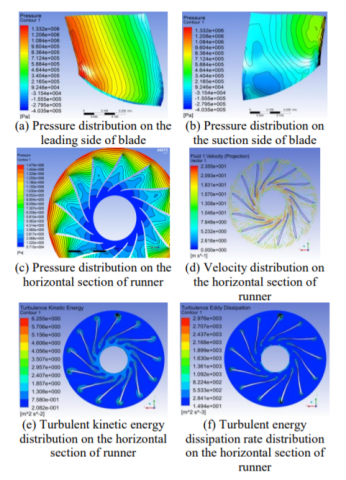Figure 3. Flow conditions in clear water

4.2 In sandy water

Figure 4 and Figure 5 show the distributions of pressure and sand concentration on the blade surface in average solid volume fractions of 0.5 % and 5 % at the case inlet, as well as the distributions of sand velocity, water velocity, turbulent kinetic energy and its dissipation rate on the horizontal section of the runner at 0.5 times the height of the blade inlet.

4.2.1 Pressure distribution on the blade surface

It can be seen from Figure 4(a, b) and Figure 5(a, b) that the changes of pressure distributions on the blade surface in sandy water and clear water were small in general, so the influence of solid volume fraction to pressure distribution was small. However, increasing solid volume fraction caused the pressure difference on the leading side and the suction side of the blade to increase, which aggravates the cavitation tendency.

## 4a.png## 4b.png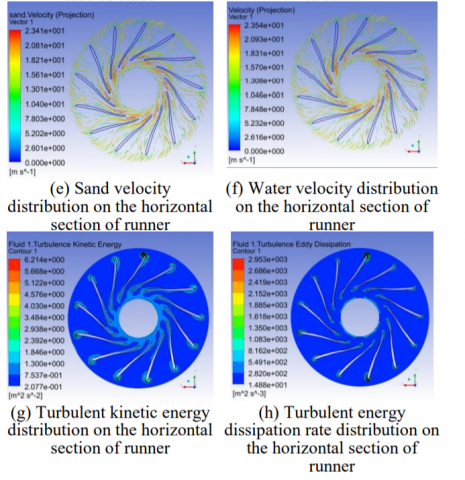Figure 4. Flow conditions in sandy water with the average solid volume fraction of 0.5%

4.2.2 Distribution of sand concentration on the blade surface

## 5a.png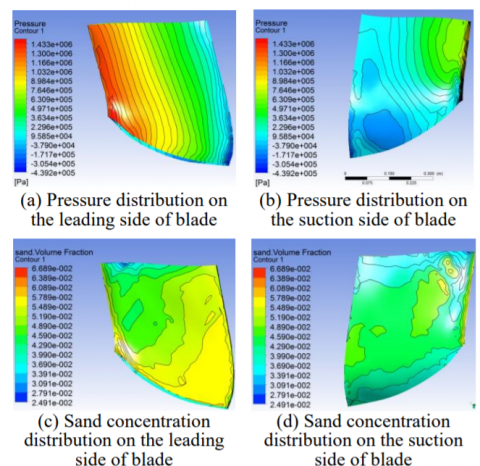## 5b.png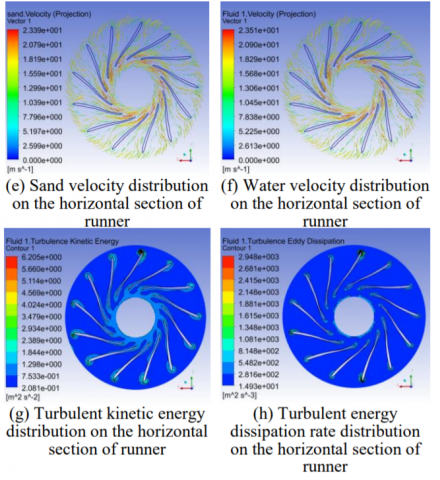Figure 5. Flow conditions in sandy water with the average solid volume fraction of 5%

With increasing solid volume fractions, the sand concentrations on the leading side and the suction side of the blade increased simultaneously. Furthermore, the variation trend of sand concentration distribution on the leading side of the blade was basically the same as that of the suction side of the blade. This means that the larger the solid volume fraction is, the worse the erosion of the entire leading side of the blade will be, starting from the outlet area near the runner band.

4.2.3 Velocity distribution in the blade channel

The influence of solid volume fraction on the velocities of sand particles and water was small, and the variation trend of velocity distribution was basically consistent. However, the velocity of sand particles was slightly less than that of the water. With increasing solid volume fractions, the velocity of sand particles fell even lower than the velocity of water, which will lead to flow separation and reduce the turbine performance.

4.2.4 Turbulent kinetic energy and its dissipation rate distribution in the runner

It can be seen from Figure 3(e, f), Figure 4(g, h) and Figure 5(g, h) that the influence of solid volume fraction on the turbulent kinetic energy and its dissipation rate distribution was small, which made it basically the same in clear water and sandy water.

5. Conclusions

By establishing a mathematical model, the solid-liquid two-phase flow conditions in a Francis turbine was simulated to study the influence of solid volume fractions on turbine performance. The following results were obtained.

(1) The influence of solid volume fractions on the pressure distributions on the blade surfaces was small, but the pressure differences on the leading side and suction side increased with solid volume factions, which will increase the cavitation tendency and reduce the turbine’s performance.

(2) The concentration of sand on the leading side was much higher than that of the suction side, so the leading side endures most of the sand erosion. On the leading side, the concentration of sand gradually increased from the inlet to the outlet, so the concentration in the area near the blade outlet and runner band was the largest. This means that the outlet area of the blade on the leading side near the runner band will be eroded first. On the suction side, the change of sand concentration was small. The distribution of sand concentration increased with the solid volume fraction both on the leading side and suction side, so the larger the solid volume fraction, the greater the erosion, and the poorer the stability of the turbine.

(3) The velocities of sand particles and water near the suction side of the blade were higher than those near the leading side of the blade. With the increasing solid volume fraction, the velocities of sand particles and water changed slightly, but the velocity of the sand particles was less than that of the pure water, therefore higher volume fractions inhibit the transfer of energy and lower the efficiency of the turbine.

(4) The turbulent kinetic energy and its dissipation rate barely changed with the increasing solid volume fraction, so the solid volume fraction does not affect the turbulent intensity.

Acknowledgment

This work was funded by the Open Research Subject of Key Laboratory of Fluid and Power Machinery (Xihua University), Ministry of Education (Grant No. szjj2015-026).

Nomenclature
 B dimensionless interphase friction coefficient d particle diameter of solid phase, mm g gravitational acceleration, m.s-2 P p time averaged pressure, Pa fluctuating pressure, Pa V time averaged velocity, m.s-1 v fluctuating velocity, m.s-1 Greek symbols δ dimensionless Kronecker delta ε dimensionless turbulent energy dissipation rate of liquid phase κ turbulent kinetic energy of the liquid phase, J ν material kinematic viscousity, kg. m-1.s-1 ρ material density, kg.m-3 σ dimensionless turbulent Schmidt number Τ fluid Largangian integral time, s τ particle response time, s $\phi$ dimensionless solid volume fraction φ dimensionless time averaged volume fraction Subscripts f liquid phase i tensor j tensor k tensor s solid phase t time
References

 B. S. Thapa, O. G. Dahlhaug and B. Thapa, “Sediment erosion in hydro turbines and its effect on the flow around guide vanes of Francis turbine,” Renewable and Sustainable Energy Reviews, vol. 49, pp. 1100-1113, Sep., 2015. DOI: 10.1016/j.rser.2015.04.178.

 B. S. Thapa, B. Thapa and O. G. Dahlhaug, “Empirical modelling of sediment erosion in Francis turbines,” Energy, vol. 41, no. 1, pp. 386-391, May, 2012. DOI: 10.1016/j.energy.2012.02.066.

 X. Liu, Y. Luo and Z. Wang, “A review on fatigue damage mechanism in hydro turbines,” Renewable and Sustainable Energy Reviews, vol. 54, pp. 1-14, Feb., 2016. DOI: 10.1016/j.rser.2015.09.025.

 M. Rashad, X. Zhang and H. Elsadek, “Numerical simulation of two-phase flow modeling of solid propellant combustion,” International Journal of Heat & Technology, vol. 32, no. 1-2, pp. 111-118, July, 2014.

 G. Peng, Z. Wang, Y. Xiao, et al., “Abrasion predictions for Francis turbines based on liquid-solid two-phase fluid simulations,” Engineering Failure Analysis, vol. 33, no. 7 , pp. 327-335, Oct., 2013. DOI: 10.1016/j.engfailanal.2013.06.002.

 H. Bounaouara, H. Ettouati, H. B. Ticha, et al., “Numerical simulation of gas-particles two phase flow in pipe of complex geometry: Pneumatic conveying of olive cake particles toward a dust burner,” International Journal of Heat & Technology, vol. 33, no. 1, pp. 99-106, 2015. DOI: 10.18280/ijht.330114.

 Q. Li, R. Li, H. Quan, et al., “Solid-liquid two-phase flow numerical simulation around guide vanes of mixed-flow water turbine,” Procedia Engineering, vol. 31, pp. 87-91, 2012. DOI: 10.1016/j.proeng.2012.01.995.

 K. Khanal, H. P. Neopane, S. Rai, et al., “A methodology for designing Francis runner blade to find minimum sediment erosion using CFD,” Renewable Energy, vol. 87, pp. 307-316, Mar., 2016. DOI: 10.1016/j.renene.2015.10.023.

 A. Mansouri, H. Arabnejad, S. Karimi, et al., “Improved CFD modeling and validation of erosion damage due to fine sand particles,” Wear, vol.338, pp. 339-350, Sep., 2015. DOI: 10.1016/j.wear.2015.07.011.

 T. Takagi, T. Okamura and J. Sato, “Hydraulic performance of a Francis-turbine for sediment-laden flow,” Hitachi Review, vol. 37, no. 2, pp. 115-120, 1998.

 H. Keck, R. Dekumbis, M. Sick, et al., “Sediment erosion in hydraulic turbines and experiences with advanced coating technologies,” in Proc. India Hydro, New Dehli, India, Feb. 19-21, 2005, pp. 74-86.

 P. J. Dunstan and S. C. Li. “Cavitation enhancement of silt erosion: numerical studies,” Wear, vol. 268, no. 7-8, pp. 946-954, Mar., 2010. DOI: 10.1016/j.wear.2009.12.036.

 R. Schilling and M. Frobenius, “Numerical simulation of the two-phase flow in centrifugal pump impellers,” in ASME 2002 Joint U.S.-European Fluids Engineering Division Conference, Montreal, Canada, July 14-18, 2002, pp. 859-865. DOI: 10.1115/FEDSM2002-31193.

 S. Chitrakar, H. P. Neopane and O. G. Dahlhaug, “Study of the simultaneous effects of secondary flow and sediment erosion in Francis turbines,” Renewable Energy, vol. 97, pp. 881-891, Nov., 2016. DOI: 10.1016/j.renene.2016.06.007.

 M. Eltvik, O. G. Dahlhaug and H. P. Neopane, “Prediction of sediment erosion in Francis turbines,” in 4th International Meeting on Cavitation and Dynamic Problems in Hydraulic Machinery and Systems, IAHR, Brisbane, Australia, June 26, 2011. URN: urn:nbn:no:ntnu:diva-22748.

 X. B. Liu and L. J. Cheng, “A k-ε two-equation turbulence model for the solid-liquid two-phase flows,” Applied Mathematics and Mechanics, vol. 17, no. 6, pp. 523-531, June, 1996. DOI: 10.1007/BF00119749.

 R. L. Peskin, “The diffusivity of small suspended particles in turbulent fluids,” in National Meeting AIChE, Baltimore, U.S., 1962.

 H. Hua, Y. Z. Zeng, H. Y. Wang, et al., “Numerical analysis of solid–liquid two-phase turbulent flow in Francis turbine runner with splitter blades in sandy water,” Advances in Mechanical Engineering, vol. 7, no. 3, 2015. DOI: 10.1177/1687814015573821.

 X. B. Liu, “Numerical simulation of silt abrasive erosion in hydraulic machinery,” Journal of Sichuan University of Science and Technology, vol. 2, pp. 79-84, 2000.